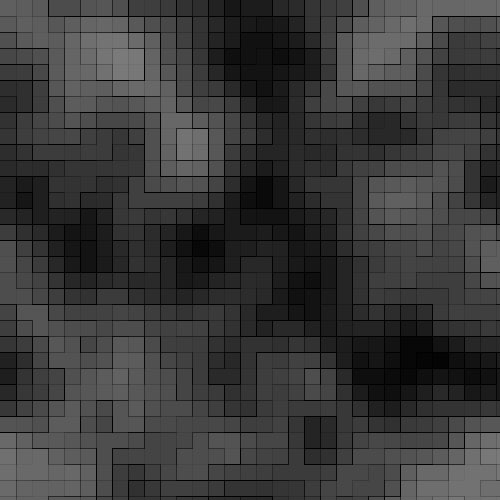# Perlin Noise

Perlin noise is most commonly implemented as a two-, three- or four-dimensional function, but can be defined for any number of dimensions.

Define an n-dimensional grid. Each grid coordinate stores a gradient of unit length in n dimensions. To sample, determine which grid cell you're in, and then compute the n-dimensional vectors from the sample location to each grid coordinate of the cell. For each grid coordinate, calculate the dot products of the corresponding distance and gradient vectors. Finally, interpolate these dot products using a function that has zero first derivative (and possibly also second derivative) at both endpoints.## Example Usage of Implementation

``````PerlinMap2D map = new PerlinMap2D(256, 10, new Random());

float arbitraryX = 765.33f;
float arbitraryY = -17;
float heightAtLocation = map.GetValueAtPoint(arbitraryX, arbitraryY);``````

## Complexity

When n is the number of dimensions, Perlin noise has complexity O(2^n), while simplex noise has complexity O(n^2).

## C# Implementation of 2D Perlin

``````public class PerlinMap2D
{
/// <summary>
/// Two dimensional perlin noise map.
/// </summary>
/// <param name="size">Total number of random points.</param>
/// <param name="subdivisions">Number of places between each point allowed to be smoothed into a gradient of all four surrounding points.</param>
/// <param name="random">The random number generator, you may pass your own if you want the same seed generation etc.</param>
public PerlinMap2D(int size, int subdivisions, Random random)
{
Size = size;
RowSize = (int)Math.Sqrt(Size);
Subdivisions = subdivisions;
Random = random;

for (int i = 0; i < Size; i++)
{
}
}

/// <summary>
/// Default recommendation. 256 Size, 10 Subdivions, new Random.
/// </summary>
public PerlinMap2D()
: this(256, 10, new Random())
{ }

/// <summary>
/// Random point generator.
/// </summary>
public Random Random;

/// <summary>
/// Total number of random points.
/// </summary>
public int Size;

/// <summary>
/// Number of places between each node allowed to be smoothed into a gradient of all four surrounding points.
/// </summary>
public int Subdivisions;

private int RowSize;
private Dictionary<int, float> NoiseMap;

/// <summary>
/// Will retrieve perlin value at any location passed.
/// </summary>
/// <param name="x">x coordinate in map</param>
/// <param name="y">y coordinate in map</param>
/// <returns></returns>
public float GetValueAtPoint(float x, float y)
{
// Locate subdivision
// X
float realX = x;
realX = realX % (RowSize * Subdivisions);
while (realX < 0)
{
realX += (RowSize * Subdivisions);
}

float perlinX = (int)(realX / Subdivisions);
perlinX = perlinX % RowSize;
while (perlinX < 0)
{
perlinX += RowSize;
}

float tX = realX - (perlinX * Subdivisions);
tX = tX / Subdivisions;

// Y
float realY = y;
realY = realY % (RowSize * Subdivisions);
while (realY < 0)
{
realY += (RowSize * Subdivisions);
}

float perlinY = (int)(realY / Subdivisions);
perlinY = perlinY % RowSize;
while (perlinY < 0)
{
perlinY += RowSize;
}

float tY = realY - (perlinY * Subdivisions);
tY = tY / Subdivisions;

// Smooth step subdivisions value.
tX = Smoothstep(tX);
tY = Smoothstep(tY);

float perlinMinX = perlinX;
float perlinMinY = perlinY;

float perlinMaxX = (perlinX + 1) % RowSize; while (perlinMinX < 0) { perlinMinX += RowSize; }
float perlinMaxY = (perlinY + 1) % RowSize; while (perlinMinY < 0) { perlinMinY += RowSize; }

float randomAt00 = NoiseMap[(int)perlinMinY * RowSize + (int)perlinMinX];
float randomAt10 = NoiseMap[(int)perlinMinY * RowSize + (int)perlinMaxX];
float randomAt01 = NoiseMap[(int)perlinMaxY * RowSize + (int)perlinMinX];
float randomAt11 = NoiseMap[(int)perlinMaxY * RowSize + (int)perlinMaxX];

float nx0 = MathHelper.Lerp(randomAt00, randomAt10, tX);
float nx1 = MathHelper.Lerp(randomAt01, randomAt11, tX);

float tTotal = MathHelper.Lerp(nx0, nx1, tY);

return tTotal;
}

private float Smoothstep( float t )
{
return t * t * ( 3 - 2 * t );
}

}``````